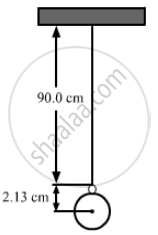Department of Pre-University Education, KarnatakaPUC Karnataka Science Class 11

# The Length of the String of a Simple Pendulum is Measured with a Metre Scale to Be 90.0 Cm. the Radius of the Bod Plus the Length of the Hook is Calculated to Be 2.13 Cm Using Measurements with - Physics

Sum

The length of the string of a simple pendulum is measured with a metre scale to be 90.0 cm. The radius of the bod plus the length of the hook is calculated to be 2.13 cm using measurements with a slide callipers. What is the effective length of the pendulum? (The effective length is defined as the distance between the point of suspension and the centre of the bob.)

#### Solution

Consider the figure shown below:Actual effective length = (90.0 + 2.13) cm
However, in the measurement 90.0 cm, the number of significant digits is only two.
So, the effective length should contain only two significant digits.
i.e., effective length = 90.0 + 2.13 = 92.1 cm.

Concept: Nature of Physical Laws
Is there an error in this question or solution?

#### APPEARS IN

HC Verma Class 11, Class 12 Concepts of Physics Vol. 1
Chapter 2 Physics and Mathematics
Exercise | Q 35 | Page 30
Share